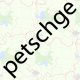# calculate bbox from lat+lon+zoom+pixel width+pixel height

 4 1 How do you calculate a bounding box (that can be used where ever a `bbox` query parameter is required) using the center longitude and latitude, the zoom factor and the displayed width and height in pixels? Basically I need this to calculate the embedding and mapnik image export URLs from a permalink. asked 16 Feb '11, 03:59panzi 66●1●2●4 accept rate: 0%

 4 Convert the longitude and latitude of the map center to tile coordinates using the alogrithm described in the Slippy Map Tilenames article on the wiki, keeping the fractional part. Add / substract half of the width from tileX and half of the height from tileY to generate the coordinates of the four corners of the bbox. Convert them back to longitude and latitude using the description from the same wiki page. All you are now left to do is to create the string describing the bbox from the coordinates of the four corners. For mapnik this should be minlong,minlat,maxlong,maxlat. answered 16 Feb '11, 07:31petschge 8.3k●21●73●98 accept rate: 21%Frederik Ramm ♦ 82.2k●92●718●1268 http://pastebin.com/mMKrhA4D is what I did with what you wrote. But the calculated values are too large. Why is that? (27 Jul '12, 22:19) Richart
 0 You probably need to take in account pixel size of each tile... I've needed this myself so this is what I've come up with and documented at OSM Wiki: ``````use Math::Trig; sub getLonLat { my (\$xtile, \$ytile, \$zoom) = @_; my \$n = 2 ** \$zoom; my \$lon_deg = \$xtile / \$n * 360.0 - 180.0; my \$lat_deg = rad2deg(atan(sinh(pi * (1 - 2 * \$ytile / \$n)))); return (\$lon_deg, \$lat_deg); } # convert from permalink OSM format like: # http://www.openstreetmap.org/?lat=43.731049999999996&lon=15.79375&zoom=13&layers=M # to OSM "Export" iframe embedded bbox format like: # http://www.openstreetmap.org/export/embed.html?bbox=15.7444,43.708,15.8431,43.7541&layer=mapnik sub LonLat_to_bbox { my (\$lat, \$lon, \$zoom) = @_; my \$width = 425; my \$height = 350; # note: must modify this to match your embed map width/height in pixels my \$tile_size = 256; my (\$xtile, \$ytile) = getTileNumber (\$lat, \$lon, \$zoom); my \$xtile_s = (\$xtile * \$tile_size - \$width/2) / \$tile_size; my \$ytile_s = (\$ytile * \$tile_size - \$height/2) / \$tile_size; my \$xtile_e = (\$xtile * \$tile_size + \$width/2) / \$tile_size; my \$ytile_e = (\$ytile * \$tile_size + \$height/2) / \$tile_size; my (\$lon_s, \$lat_s) = getLonLat(\$xtile_s, \$ytile_s, \$zoom); my (\$lon_e, \$lat_e) = getLonLat(\$xtile_e, \$ytile_e, \$zoom); my \$bbox = "\$lon_s,\$lat_s,\$lon_e,\$lat_e"; return \$bbox; } `````` You can see code in action here answered 10 Jun '13, 18:08Matija Nalis 750●2●9●16 accept rate: 11%
 toggle preview community wiki:

By Email:

Markdown Basics

• *italic* or _italic_
• **bold** or __bold__
• image?![alt text](/path/img.jpg "title")
• numbered list: 1. Foo 2. Bar
• to add a line break simply add two spaces to where you would like the new line to be.
• basic HTML tags are also supported

Question tags:

×33
×29
×10
×8

question asked: 16 Feb '11, 03:59

question was seen: 17,031 times

last updated: 14 Jun '13, 15:53